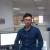## 继续是修饰以及函数返回值的问题

ka-keung 发布于 2014/11/27 21:57

```def first(A):
def one(B):
print ctime(),A(B)
return one

def sec(C):
def two(D):
x='XXXXXXXXX',C(D)
print x
return two

@sec
@first
def test(w):
return 'hello %s' %w```

>>> test(9)
Thu Nov 27 21:52:30 2014 hello 9
('XXXXXXXXX', None)

0test函数有返回值，你的one和two对test进行调用后不将其返回值返回，相当于重新改造了test函数的功能，从一个‘修饰链'变成了‘拦截器’。

```def first(A):
def one(B):
ret = A(B)
print ctime(),ret
return ret
return one

def sec(C):
def two(D):
ret = C(D)
x='XXXXXXXXX', ret
print x
return ret
return two

@sec
@first
def test(w):
return 'hello %s' %w```